Next: Output of Fourier Transforms Up: Storage: What Goes Where Previous: Storage: What Goes Where

### Fourier Transform Definitions and Conventions

The Fourier transform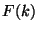of a function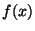is defined as(1)(2)

A discrete Fourier transform takes a functionknown only atdiscrete pointsand gives back a functionknown only at the discrete points(3)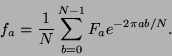(4)

Note that the relationship between the discrete Fourier transform and the continuous one is(5)

where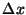is the spacing between pointsand the spacing between frequenciesis given by(6)

(Note that I am following the conventions of Numerical Recipes using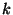to denote frequency rather than angular frequency. The conversion is simply.) Formally the discrete Fourier transformis periodic with periodsocan take any set ofconsecutive values. Practically speaking, though, if the pointsrepresent a typical region of the functionthen the resultsgive the frequency components in the range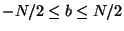(whereby periodicity). The next section describes how these complex points are arranged in the output of the routines fftc1 and fftcn. The following section describes the additional information needed to interpret the results of the real Fourier transform routines fftr1 and fftrn.Next: Output of Fourier Transforms Up: Storage: What Goes Where Previous: Storage: What Goes Where